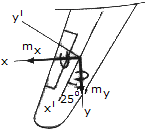# Engineering Mechanics - Force System Resultants - Discussion

### Discussion :: Force System Resultants - General Questions (Q.No.1)

1.The main beam along the wing of an airplane is swept back at an angle of 25°. From load calculations it is determined that the beam is subjected to couple moments aMx = 25,000 lb • ft and My = 17,000 lb • ft. Determine the equivalent couple moments created about the x' and y' axis.

 [A]. Mx' = 26.0 kip-ft, My' = 29.8 kip-ft [B]. Mx' = 29.8 kip-ft, My' = -4.84 kip-ft [C]. Mx' = 26.0 kip-ft, My' = 15.47 kip-ft [D]. Mx' = 29.8 kip-ft, My' = 4.84 kip-ft

Explanation:

No answer description available for this question.

 Irfan said: (Oct 11, 2015) Somebody can show steps to calculate this problem.

 Saideep said: (Oct 26, 2015) Please give me explanation answer.

 Bunny said: (Oct 10, 2017) Mx'= 25sin25+17cos25 = 25.97kN.m. My'= 25cos25-17sin25 = 15.47kN.m.